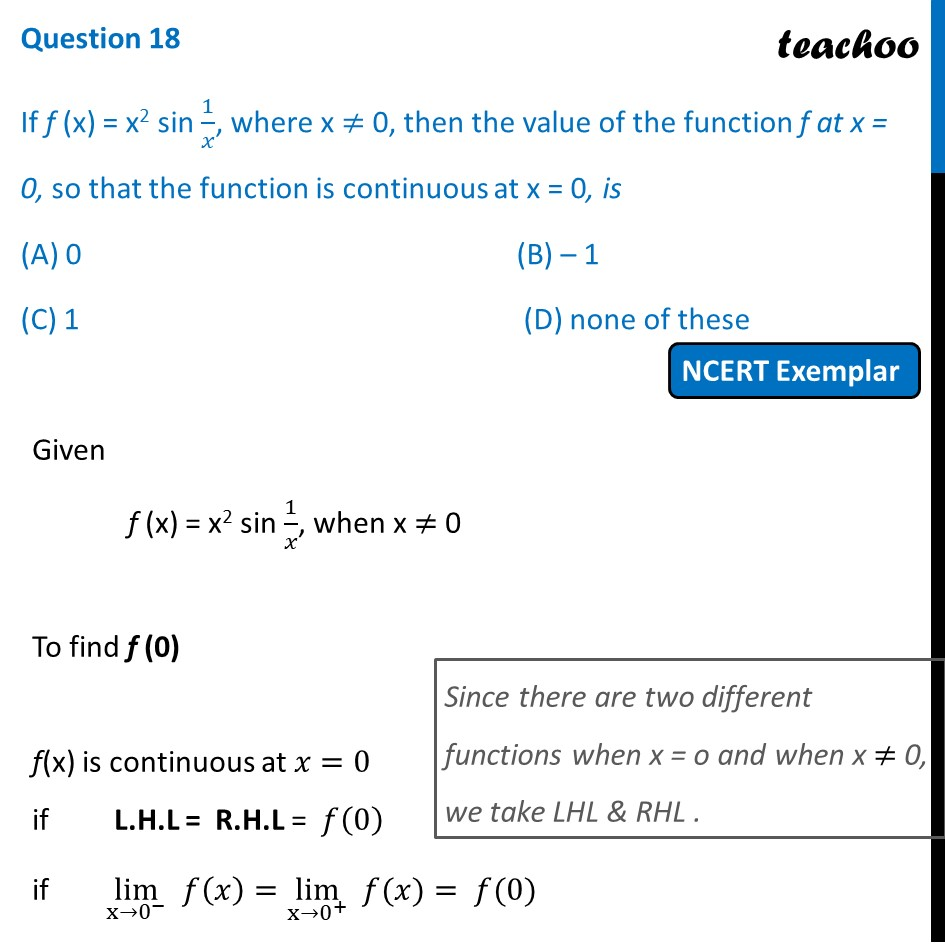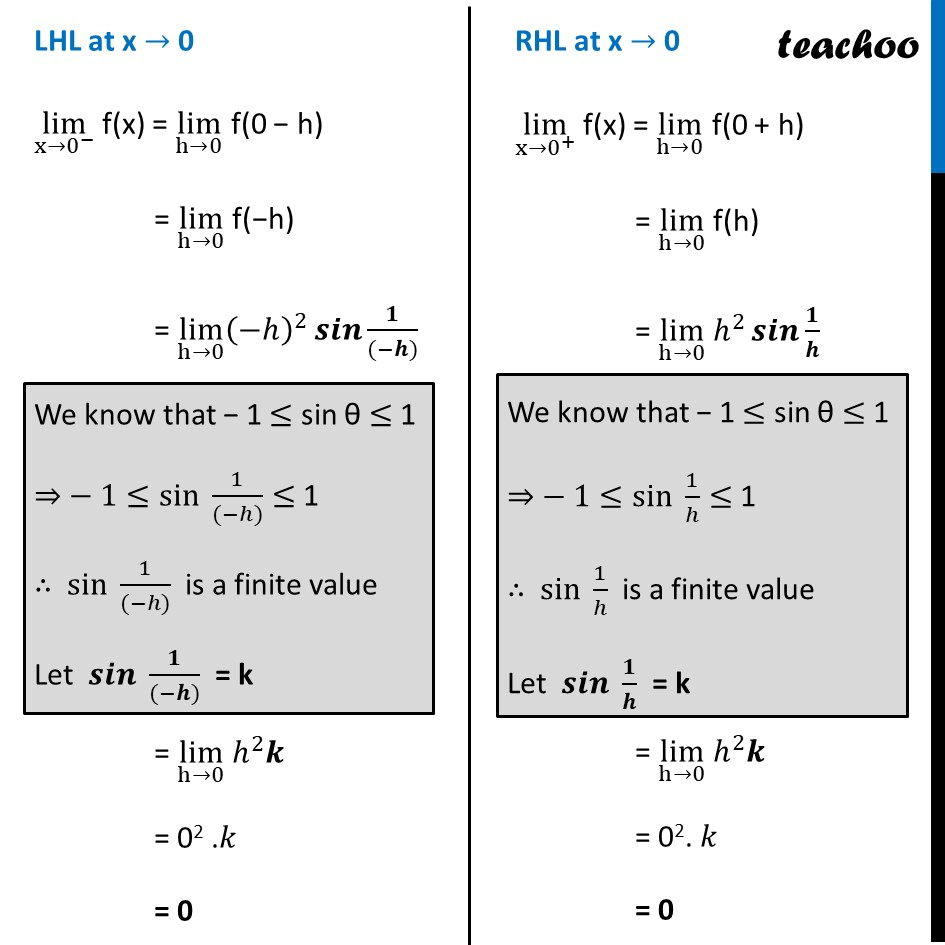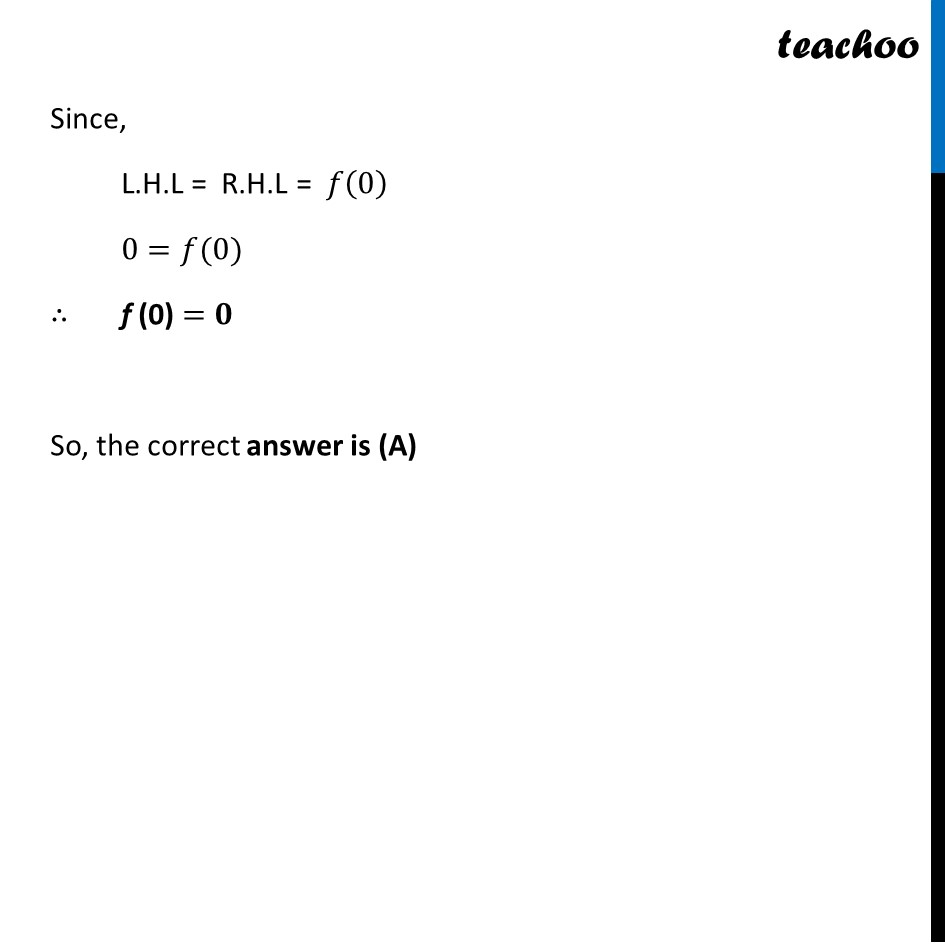NCERT Exemplar - MCQs

Chapter 5 Class 12 Continuity and Differentiability
Serial order wise

## (D) none of these

This question is similar to Ex 5.1, 24 - Chapter 5 Class 12 - Continuity and DifferentiabilityLearn in your speed, with individual attention - Teachoo Maths 1-on-1 Class

### Transcript

Question 16 If f (x) = x2 sin 1/𝑥, where x ≠ 0, then the value of the function f at x = 0, so that the function is continuous at x = 0, is (A) 0 (B) – 1 (C) 1 (D) none of these Given f (x) = x2 sin 1/𝑥, when x ≠ 0 To find f (0) f(x) is continuous at 𝑥=0 if L.H.L = R.H.L = 𝑓(0) if lim┬(x→0^− ) 𝑓(𝑥)=lim┬(x→0^+ ) " " 𝑓(𝑥)= 𝑓(0) LHL at x → 0 lim┬(x→0^− ) f(x) = lim┬(h→0) f(0 − h) = lim┬(h→0) f(−h) = lim┬(h→0) (−ℎ)^2 𝒔𝒊𝒏⁡〖𝟏/((−𝒉))〗 = lim┬(h→0) ℎ^2 𝒌 = 02 .𝑘 = 0 RHL at x → 0 lim┬(x→0^+ ) f(x) = lim┬(h→0) f(0 + h) = lim┬(h→0) f(h) = lim┬(h→0) ℎ^2 𝒔𝒊𝒏⁡〖𝟏/𝒉〗 = lim┬(h→0) ℎ^2 𝒌 = 02. 𝑘 = 0 Since, L.H.L = R.H.L = 𝑓(0) 0=𝑓(0) ∴ f (0) =𝟎 So, the correct answer is (A)